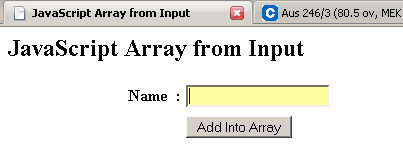Tutorials

# JavaScript Array from Input

In this Tutorial we want to describe you a code that makes you easy to understand an example of Array from Input.

In this Tutorial we want to describe you a code that makes you easy to understand an example of Array from Input.

# JavaScript Array from Input

In this Tutorial we want to describe you a code that makes you easy to understand an example of  Array from Input. The example create a HTML Page JavaScript array from input include a text name 'name', a text field and a button name inset into array. On click a button, a show( ) and insert ( ) function is invoked. The array variable instantiate an array object and hold its values in an array. The function insert ( )accept the value entered by the user. The function show ( ) includes string variable that hold all the element of the array. The for loop run and execute the script till the length i is less than array length. The variable string store the concatenated value of string and array.

The if operator return you the all the element of string in case the array length is more than zero.

JavaScript Array from Input.html

 ```       JavaScript Array from Input

JavaScript Array from Input

Name    :                                                       ```

OutputAfter insert value